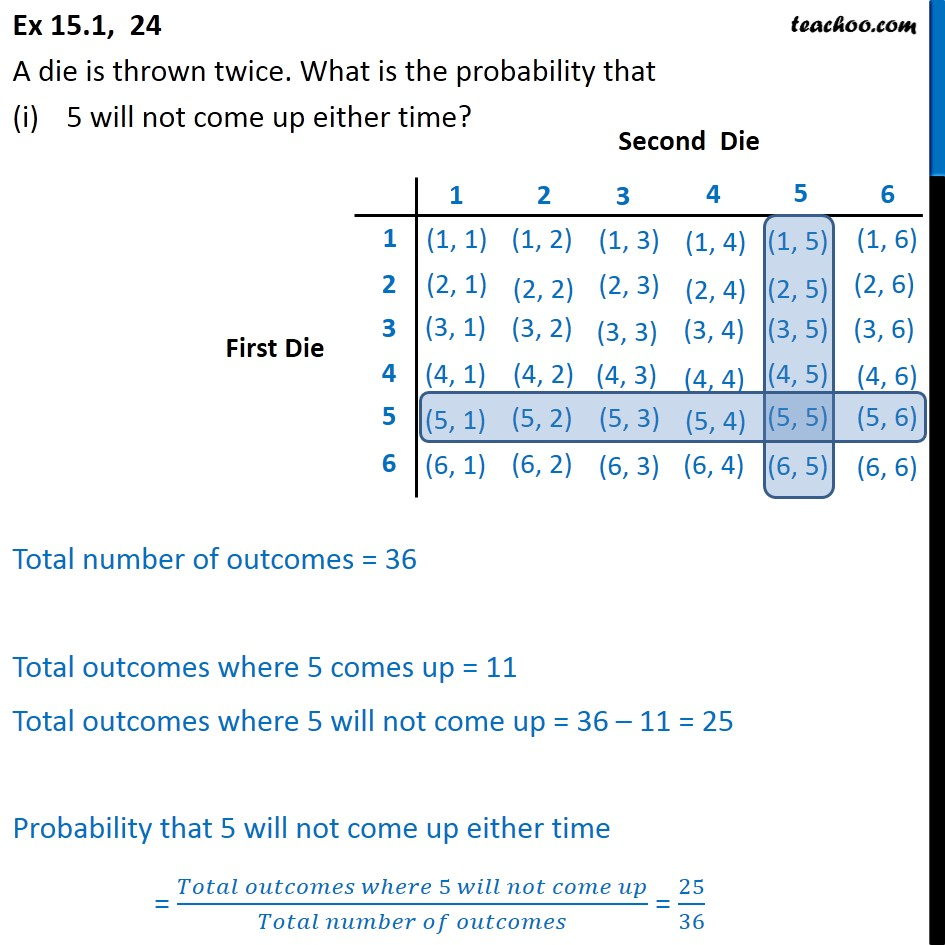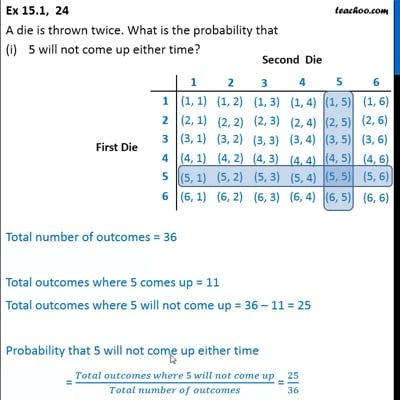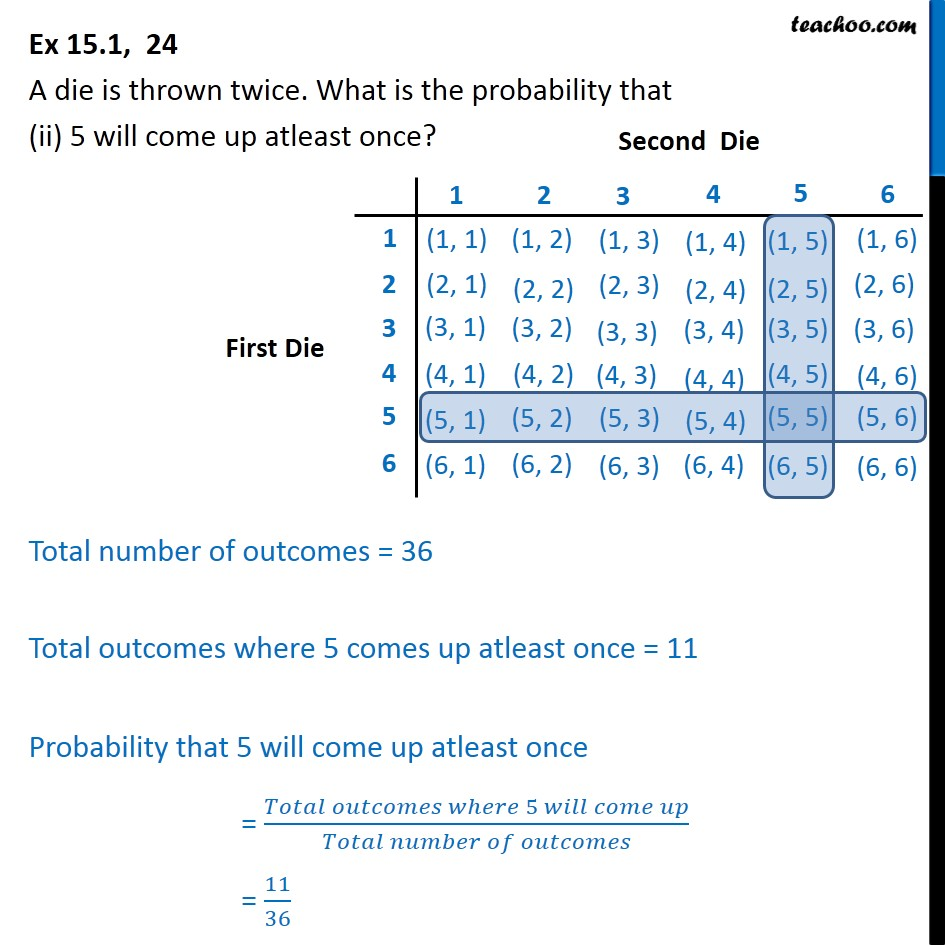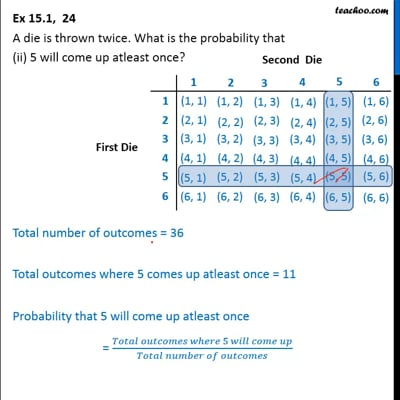Ex 15.1

Chapter 15 Class 10 Probability
Serial order wiseThis video is only available for Teachoo black usersThis video is only available for Teachoo black users

Get live Maths 1-on-1 Classs - Class 6 to 12

### Transcript

Ex 15.1, 24 A die is thrown twice. What is the probability that 5 will not come up either time? Total number of outcomes = 36 Total outcomes where 5 comes up = 11 Total outcomes where 5 will not come up = 36 – 11 = 25 Probability that 5 will not come up either time = (𝑇𝑜𝑡𝑎𝑙 𝑜𝑢𝑡𝑐𝑜𝑚𝑒𝑠 𝑤ℎ𝑒𝑟𝑒 5 𝑤𝑖𝑙𝑙 𝑛𝑜𝑡 𝑐𝑜𝑚𝑒 𝑢𝑝)/(𝑇𝑜𝑡𝑎𝑙 𝑛𝑢𝑚𝑏𝑒𝑟 𝑜𝑓 𝑜𝑢𝑡𝑐𝑜𝑚𝑒𝑠) = 25/36 Ex 15.1, 24 A die is thrown twice. What is the probability that (ii) 5 will come up atleast once? Total number of outcomes = 36 Total outcomes where 5 comes up atleast once = 11 Probability that 5 will come up atleast once = (𝑇𝑜𝑡𝑎𝑙 𝑜𝑢𝑡𝑐𝑜𝑚𝑒𝑠 𝑤ℎ𝑒𝑟𝑒 5 𝑤𝑖𝑙𝑙 𝑐𝑜𝑚𝑒 𝑢𝑝)/(𝑇𝑜𝑡𝑎𝑙 𝑛𝑢𝑚𝑏𝑒𝑟 𝑜𝑓 𝑜𝑢𝑡𝑐𝑜𝑚𝑒𝑠) = 11/36